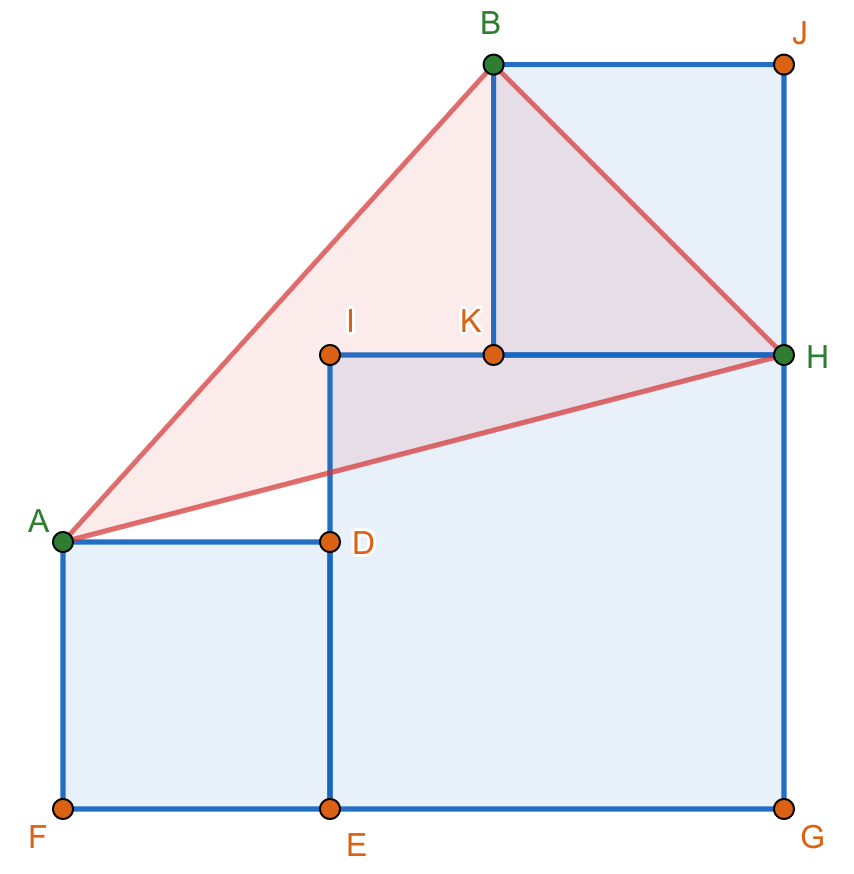# Area of Triangle## Area of a triangle

In square $ABCD$, $E$ is the midpoint of side $\overline{AB}$ and $F$ is a point of side $\overline{AD}$ such that $F$ is twice as near from $D$ as from $A$. $G$ is the intersection of the line segments $\overline{DE}$ and $\overline{CF}$. If $AB = 1\text{ cm}$, find the area of $\triangle CDG$.

There are several ways to solve this problem, please show your solutions.## Constant Area of Triangle ABC

From the figures shown below. Squares EGHI and KHJB are fixed. Show that the area of triangle ABH is constant regardless of the dimensions of square ADEF.

Figure 1Figure 2